# Mass-spring-damper system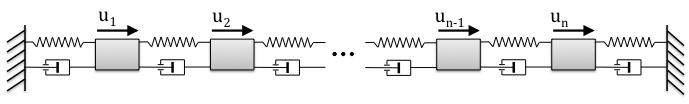For a stochastically-forced mass-spring-damper system with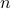masses on a line with state-space representation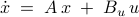the state vector is determined by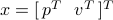and contains the position and velocity of all masses. The state and input matrices are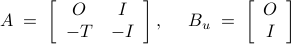where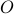and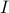are zero and identity matrices of suitable sizes, and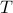is a symmetric tridiagonal Toeplitz matrix with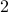on the main diagonal and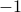on the first upper and lower sub-diagonals. Stochastic disturbances are generated by a low-pass filter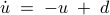where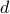represents a zero-mean unit variance white process. The steady-state covariance of the of the stochastically-forced mass-spring-damper system can be extracted from the steady-state covariance of the cascade of filter and system dynamics, and is partitioned as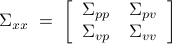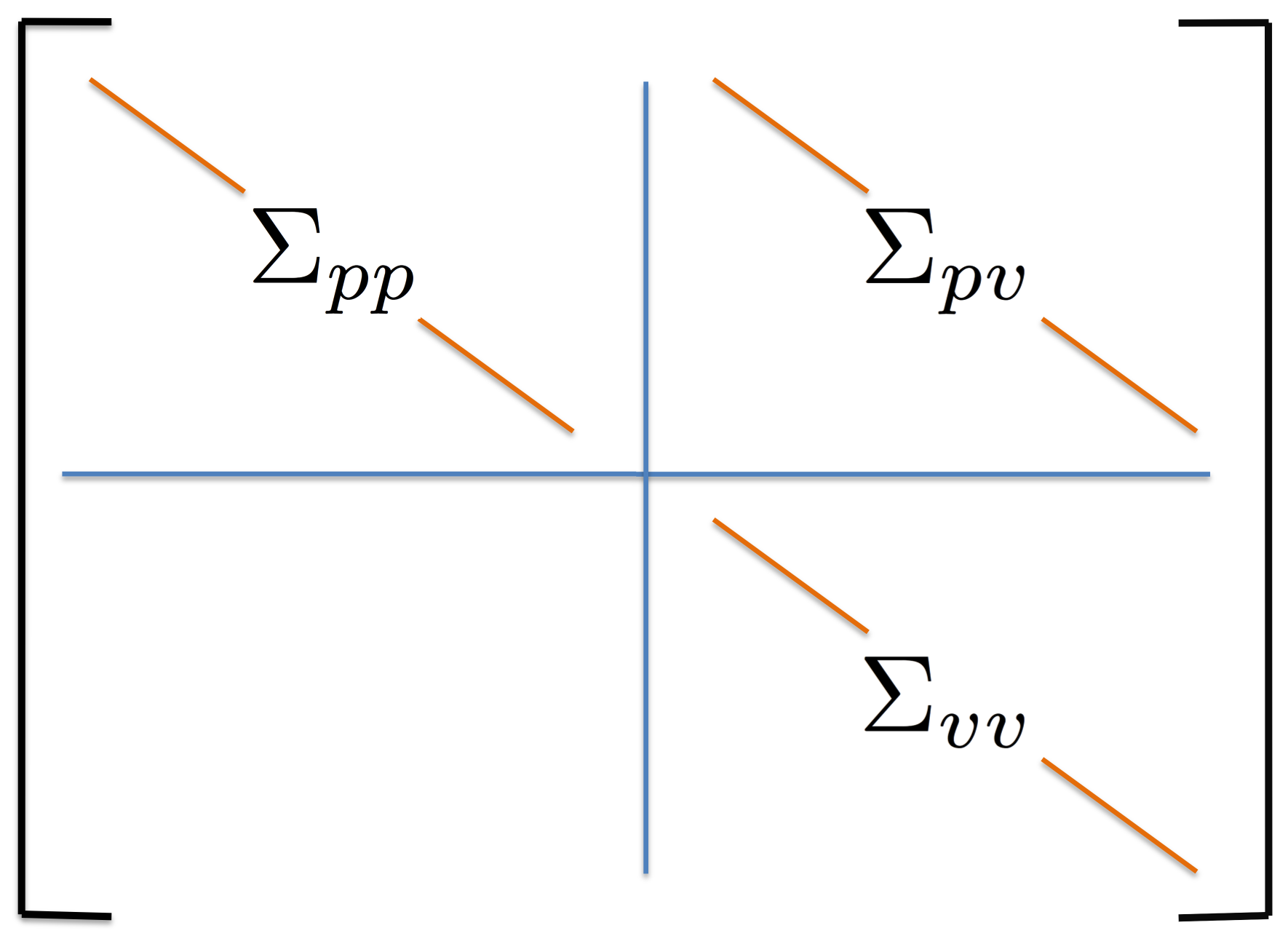We assume knowledge of one-point correlations of the position and velocity of masses, i.e., the diagonal elements of matrices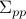,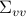, and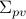, which are shown as orange lines in the figure. It is desired to identify the suitable forcing model which accounts for the available and completes the unavailable second-order statistics in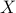.

### Performance comparison

The following table compares solve times of CVX and the customized algorithms discussed in the paper. Each method stops when an iterate achieves a certain distance from optimality, i.e.,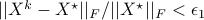and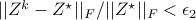. The choice of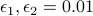, guarantees that the primal objective is within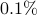of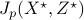. For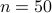and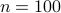, CVX ran out of memory. Clearly, for large problems, AMA with BB step-size initialization significantly outperforms both regular AMA and ADMM.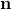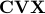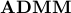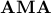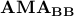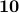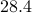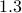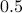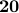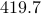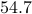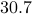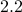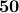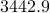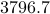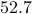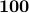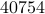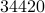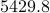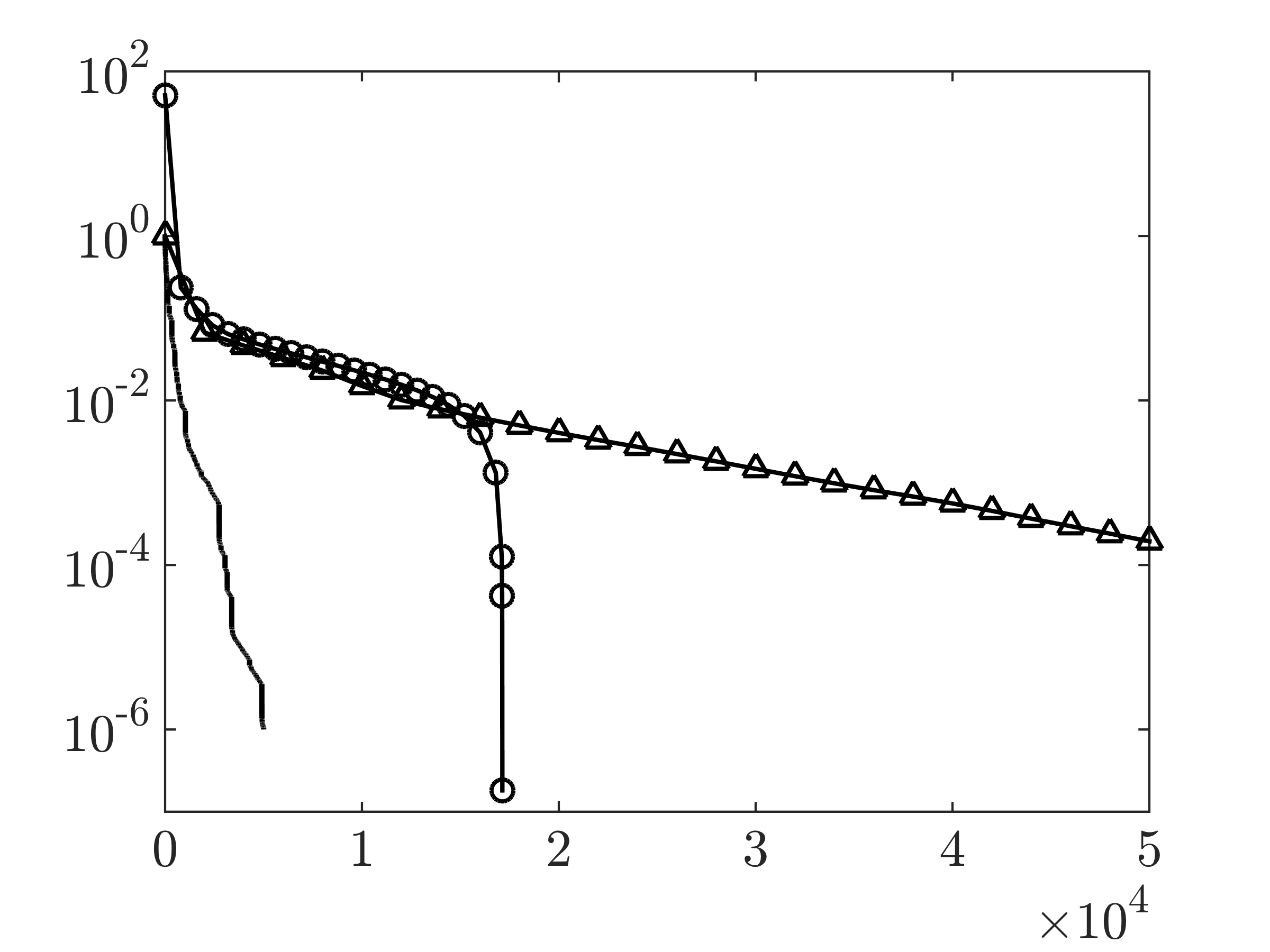Convergence curves showing the performance of the customized AMA with (-) and without (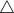) BB step-size initialization and ADMM () for the mass-spring-damper system with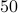masses and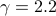. Here,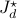is the optimal dual objective.

### Quality of completion and verification in linear stochastic simulations

For, the spectrum of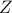containspositive and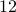negative eigenvalues. Based on Propositionof the paper,can be decomposed into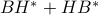, where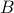hasindependent columns. In other words, the identifiedcan be explained by driving the state-space model withstochastic inputs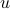. Based on this, we construct the optimal filter that generates the suitable stochastic input and conduct linear stochastic simulations of the filter dynamics with zero-mean unit variance input to validate our modeling approach.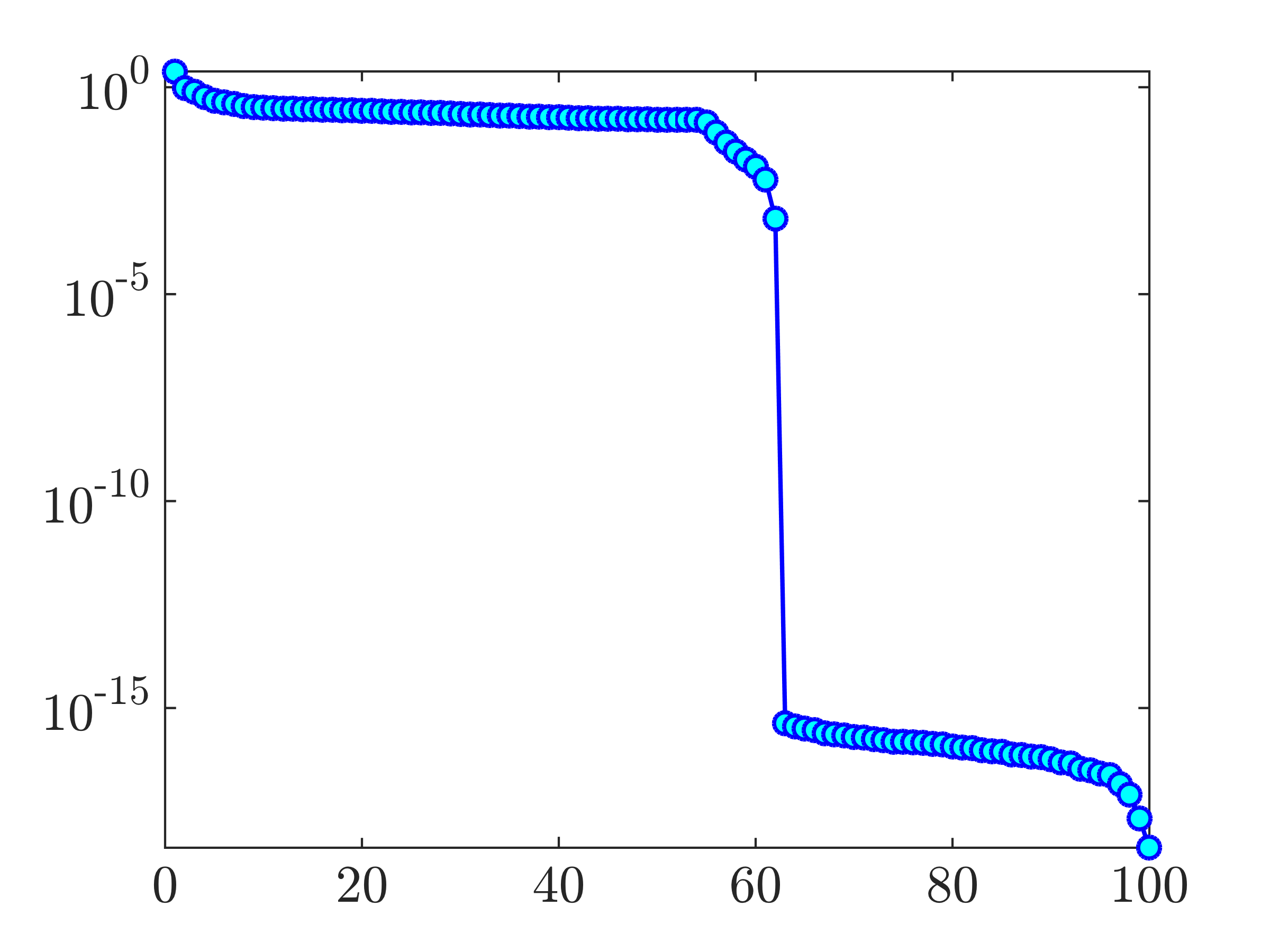For, the matrixwhich results from solving problem (CC) has a clear-cut in its singular values with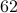of them being nonzero.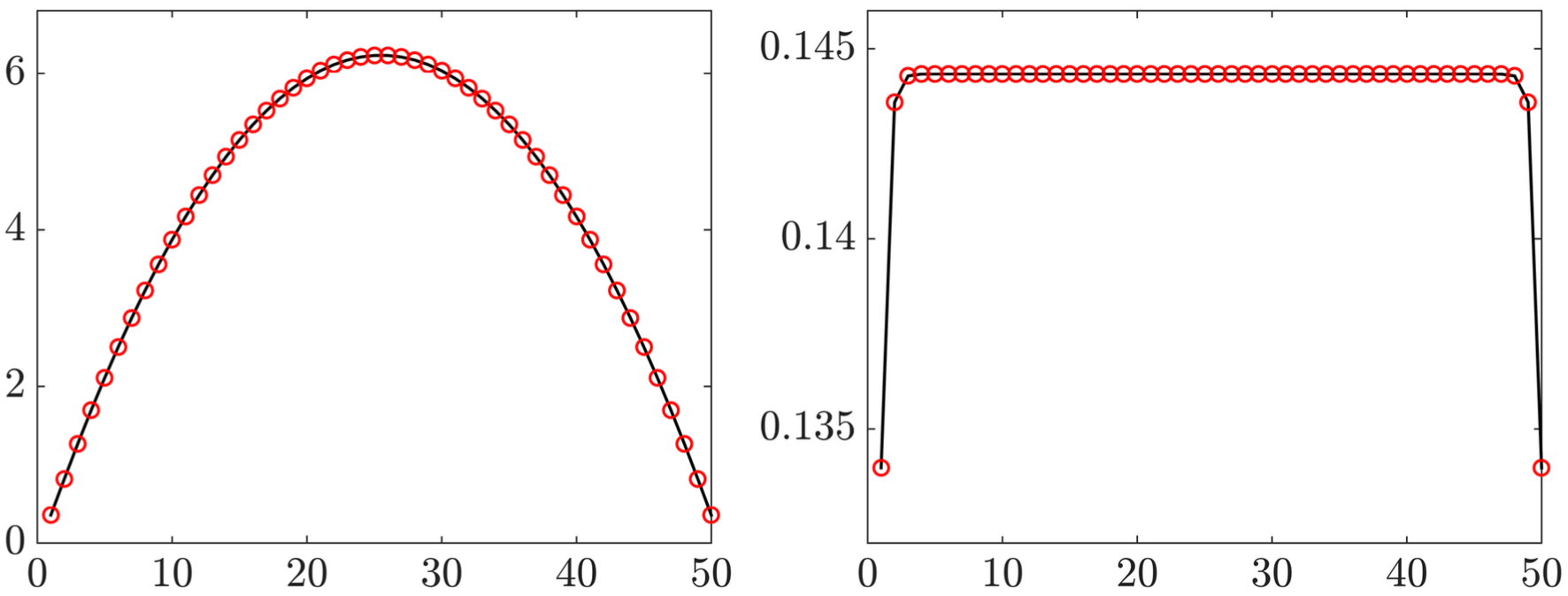Diagonals of position (left) and velocity (right) covariances for the mass-spring- damper system withmasses; Solid black lines show diagonals of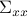and red circles mark solutions of optimization problem (CC).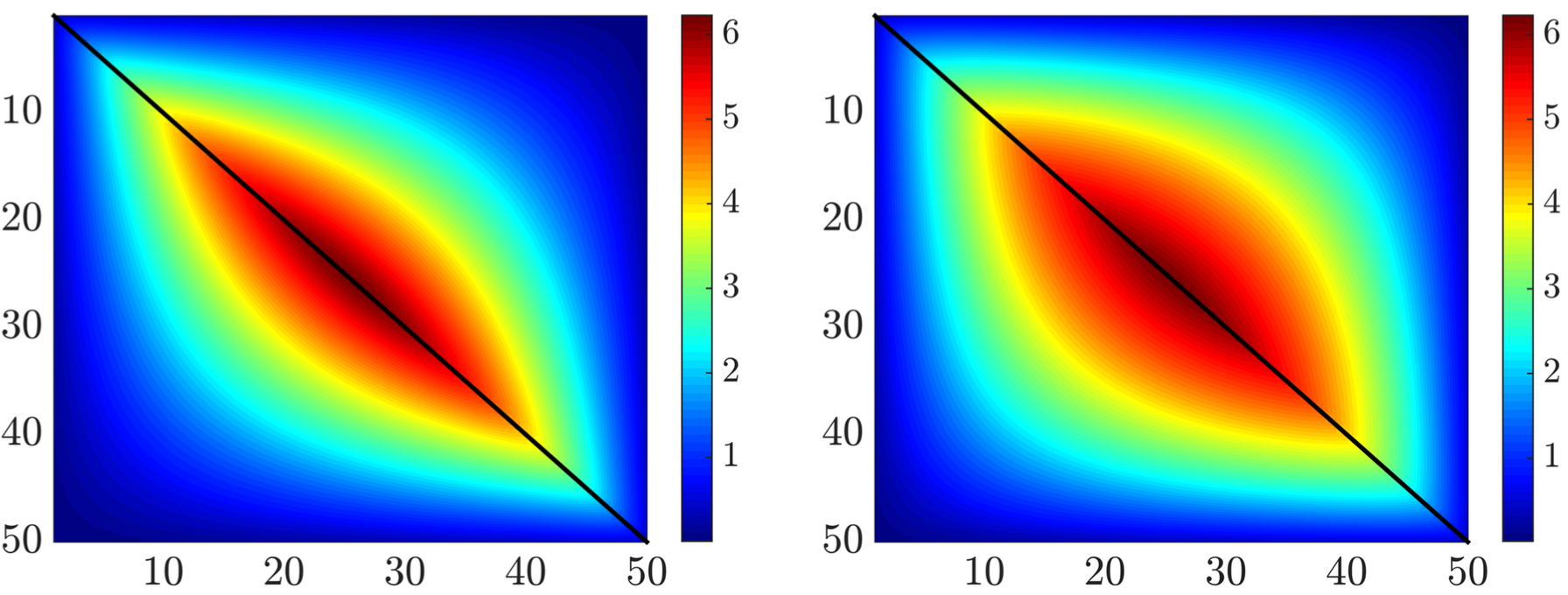The true covarianceof the mass-spring-damper system (left) and the recovered covariance matrix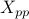resulting from the ensemble-averaged simulations (right). We note that (i) only diagonal elements of this matrix (marked by the black line) are used as data in optimization problem (CC), and that (ii) the recovery of the off-diagonal elements is remarkably consistent.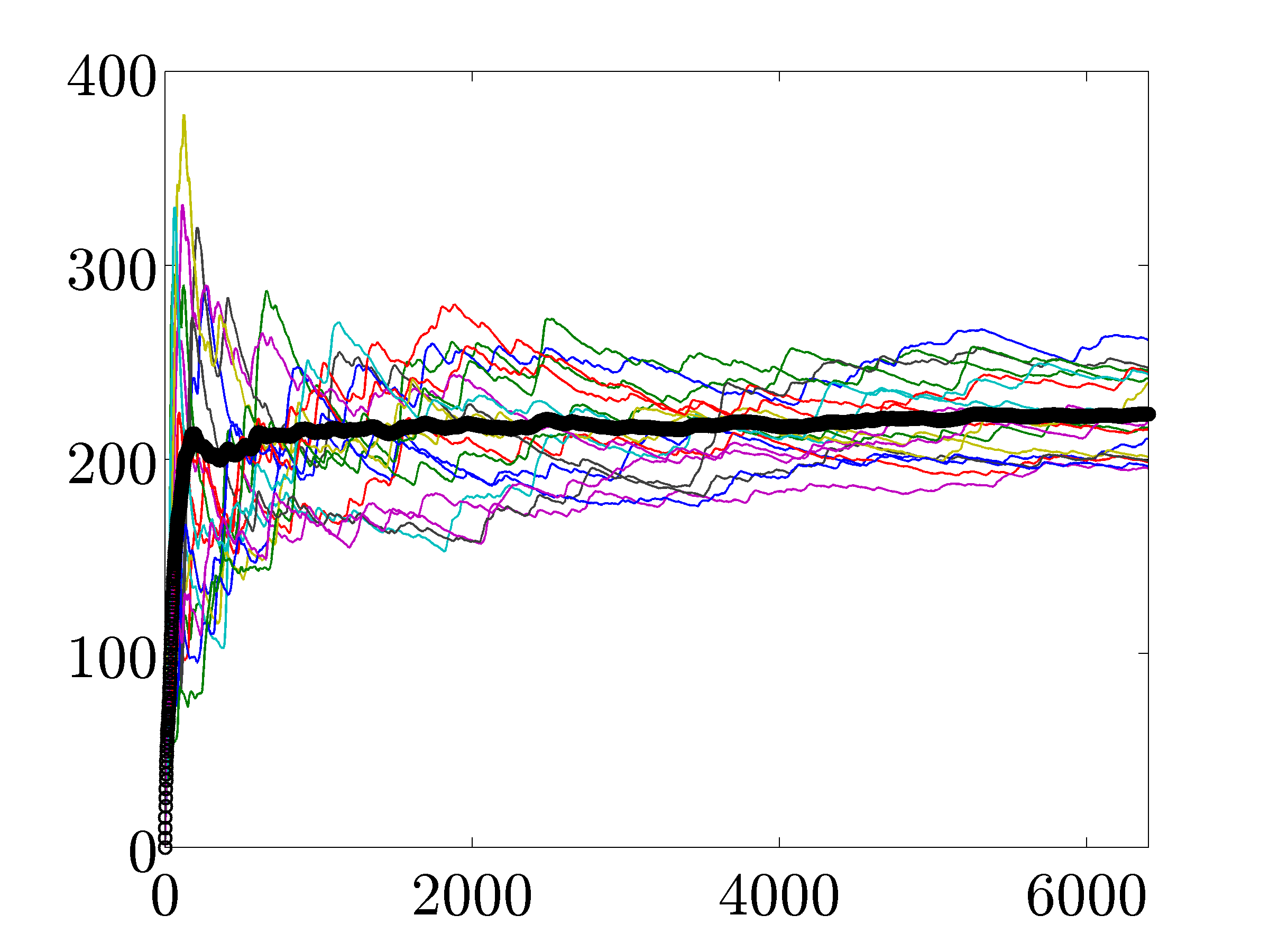Time evolution of the variance of the mass-spring-damper system's state vector for different realizations of white-in-time forcing to the filter dynamics. Since proper comparison requires ensemble-averaging, we have conducted twenty stochastic simulations with different realizations of the stochastic input. The variance averaged over all simulations is marked by the thick black line. Even though the responses of individual simulations differ from each other, the average of twenty sample sets asymptotically approaches the correct steady-state variance.